Số lượng: 0

Tổng cộng: 0,00

0

# Colouring a cube

### Colouring a cube

Colouring the vertices, edges and faces of a given cube according to the criteria specified in the exercise.

Toán học

Từ khoá

khối lập phương, mặt hàng xóm, đỉnh, cạnh lề, đối mặt, tô màu

Các mục liên quan

### Các cảnh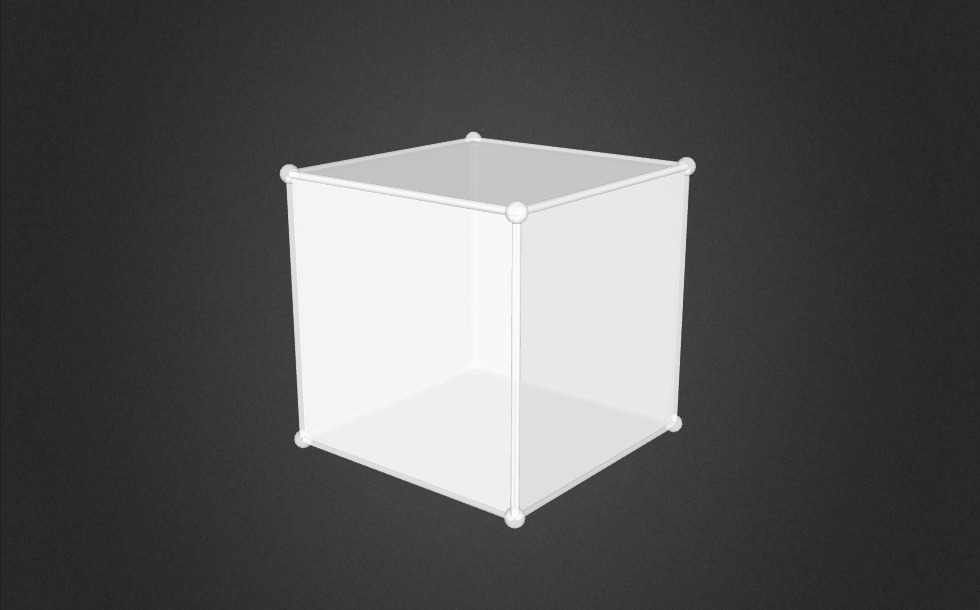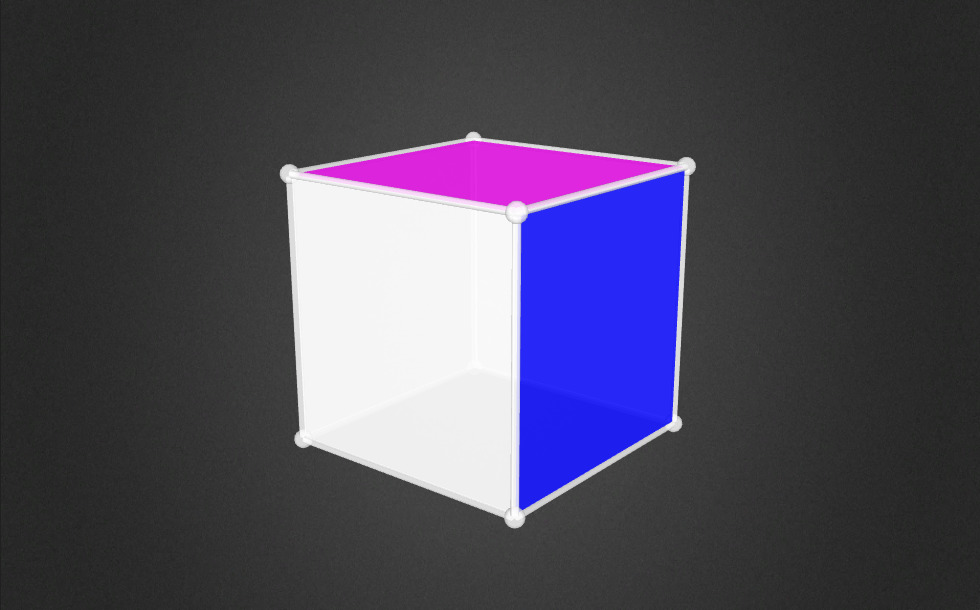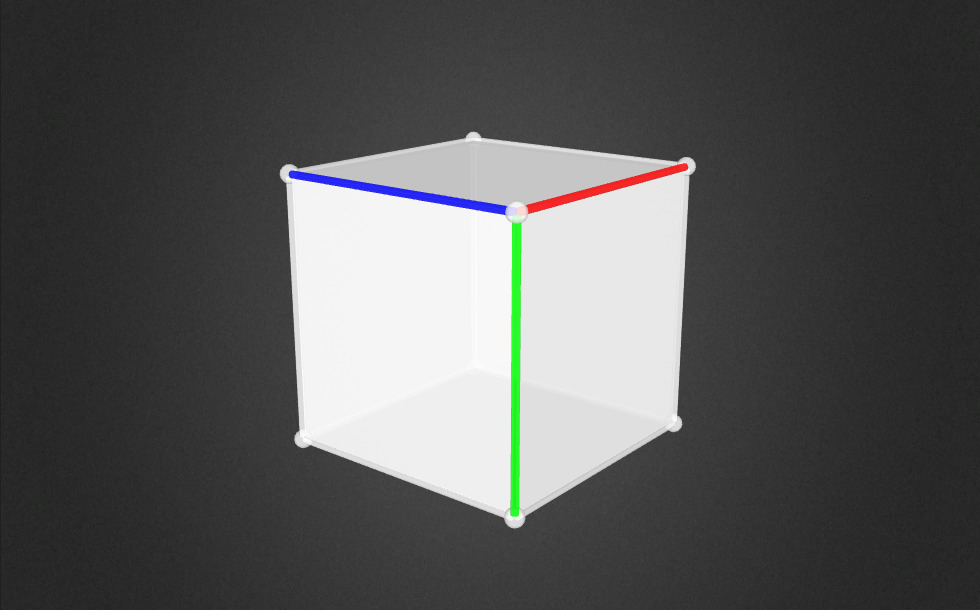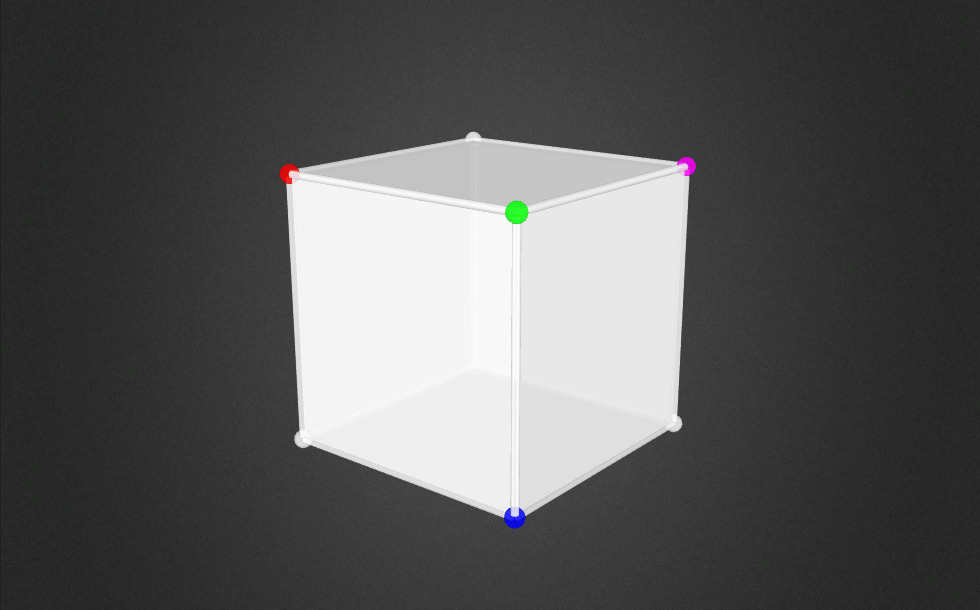### Các mục liên quan#### Cube

This animation demonstrates the components (vertices, edges, diagonals and faces) of the cube, one of the Platonic solids.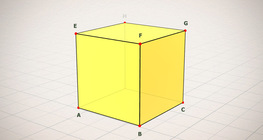#### Cube (exercises)

Edges, diagonals and faces of a cube can be identified by its vertices.#### Cube of cubes

An exercise about the regular hexahedron built from unit cubes to help deepen your knowledge of cubes.#### Cube puzzle

Building cubes shown in several views from the available unit cubes aids spatial vision and other skills.#### Cube sections (exercise)

Examining solids formed by the intersection of a cube and a plane.#### Net of a cube (exercises)

Not all nets consisting of 6 congruent squares are foldable into cubes.#### Building shapes (one colour)

Build 3D shapes from unit cubes with the help of several views.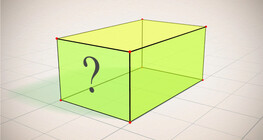#### Cuboid (exercises)

Edges, diagonals and faces of a cuboid can be identified by its vertices.#### Building shapes (multi colour)

Build 3D shapes from unit cubes with the help of several views.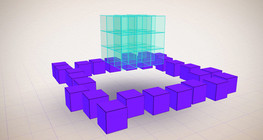#### Constructing shapes (3D)

Building 3D shapes into a given cube mesh with the help of several views.#### Cuboid

A cuboid is a polyhedron with six rectangular faces.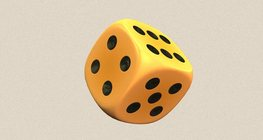#### Dice

Regular dice can be used for solving statistical and probability exercises.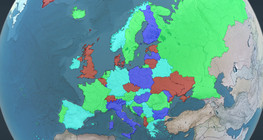#### Four colour theorem

Colour a map with the fewest number of colours possible, so that no two adjacent regions have the same colour.#### Grouping of cuboids

This animation demonstrates various types of cuboids through everyday objects.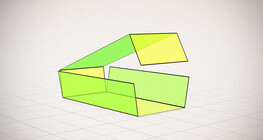#### Nets of a cuboid (exercises)

This animation demonstrates the different nets of a cuboid and includes a game.#### Notable products

A spectacular demonstration of algebraic expressions.#### Volume and surface area (exercise)

An exercise about the volume and surface area of solids generated from a ´base cube´.